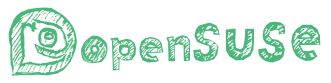# 你们都用什么工具画图？

octave:1> angles=linspace(0,2*pi,100);
octave:2> y=tan(angles);
octave:3> plot(angles,y)
octave:4> axis([0 7 -5 5])

octave 画出来比较丑啊，而且曲线也不够光滑．GeoGebra 看起来效果不错的样子．

[quote=“hottea”]octave 画出来比较丑啊，而且曲线也不够光滑．GeoGebra 看起来效果不错的样子．

octave:1> angles=linspace(0,2*pi,1000);

[/quote]

Python 的 Jupyter 交互式画图不错。如果需要写脚本就 import matplotlib 等就行了

``````def tannan(x, bound=6):
t=tan(x)
if abs(t)>bound:
return nan
else:
return t
tann=vectorize(tannan)
x=arange(0,9*pi/2,pi/100)
plot(x,tann(x))
``````

py生成的图也可以是矢量的pdf等格式。asy的话和tex搭配当然天衣无缝。

Sent from my Redmi Note 2 using Tapatalk